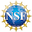Register

### 博客## The flow of polynomial roots under differentiation

Alexander Kiselev, and Changhui Tan

Abstract

The question about behavior of gaps between zeros of polynomials under differentiation is classical and goes back to Marcel Riesz. Recently, Stefan Steinerberger formally derived a nonlocal nonlinear partial differential equation which models dynamics of roots of polynomials under differentiation. In this paper, we connect rigorously solutions of Steinerberger’s PDE and evolution of roots under differentiation for a class of trigonometric polynomials. Namely, we prove that the distribution of the zeros of the derivatives of a polynomial and the corresponding solutions of the PDE remain close for all times. The global in time control follows from the analysis of the propagation of errors equation, which turns out to be a nonlinear fractional heat equation with the main term similar to the modulated discretized fractional Laplacian $$(-\Delta)^{1/2}$$.This work is supported by NSF grant DMS #1853001 and DMS #2108264

This paper is the second part of the preprint arXiv:2012.09080.